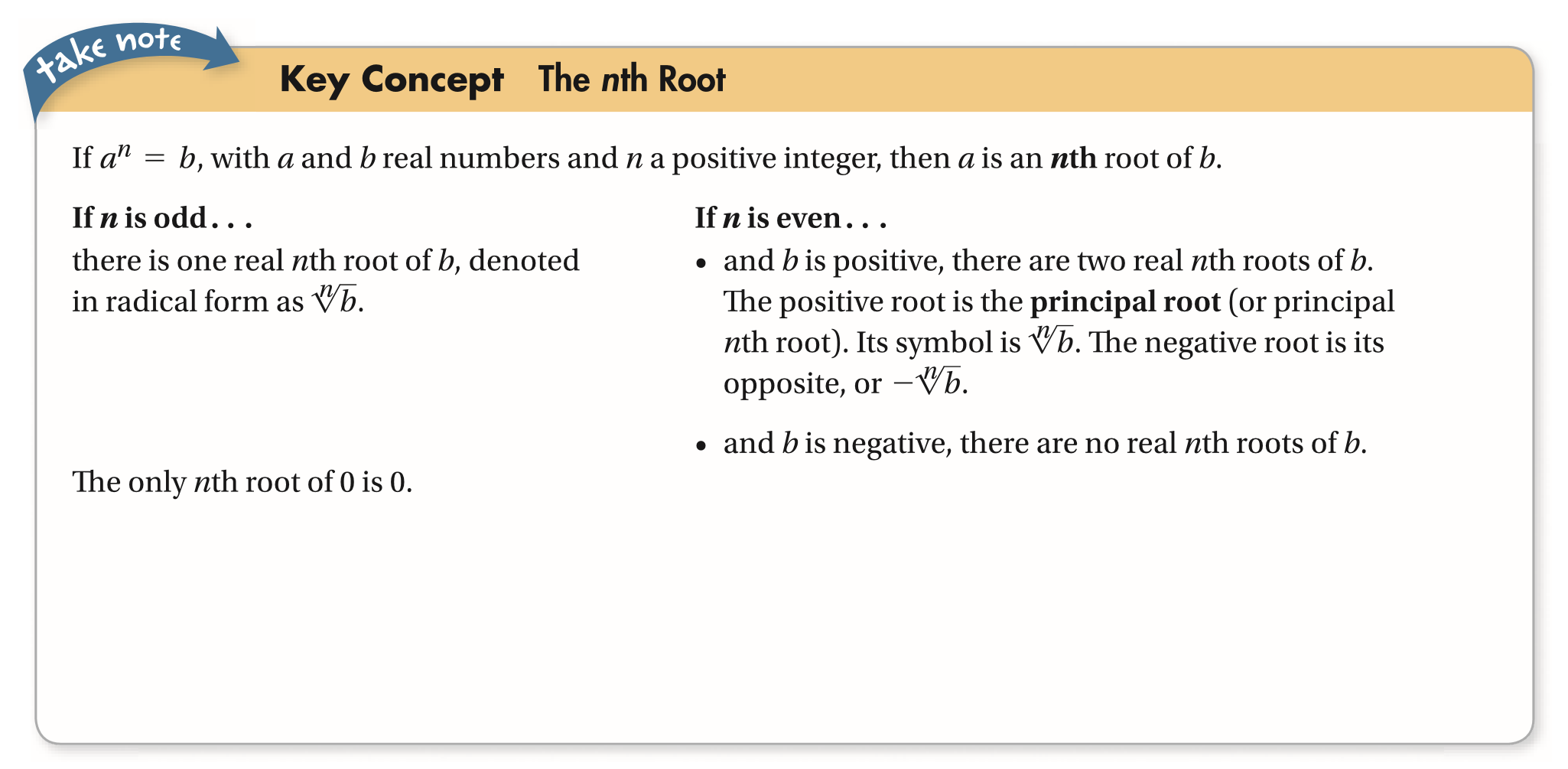Algebra 2 6-1 Complete Lesson: Roots and Radical Expressions
starstarstarstarstarstarstarstarstarstar
4.5 (1 rating)
by Matthew Richardson
| 32 Questions
Note from the author:
A complete formative lesson with embedded slideshow, mini lecture screencasts, checks for understanding, practice items, mixed review, and reflection. I create these assignments to supplement each lesson of Pearson's Common Core Edition Algebra 1, Algebra 2, and Geometry courses. See also mathquest.net and twitter.com/mathquestEDU.The outlined content above was added from outside of Formative.
1
10 pts
Solve It! This equation contains an infinite radical.
Square each side. You get a quadratic equation that also contains an infinite radical.
Are the two solutions of the quadratic equation also solutions of this equation? Explain.
Hint: consider substitution from one equation into the other.
No. By substitution:
Therefore, the solutions of the quadratic equation are both positive and negative, as follows:
The solution to the original equation is positive.
Yes. Given that
and that radicals simplify to only real numbers that are positive, as shown here:
both equations must have the same solutions.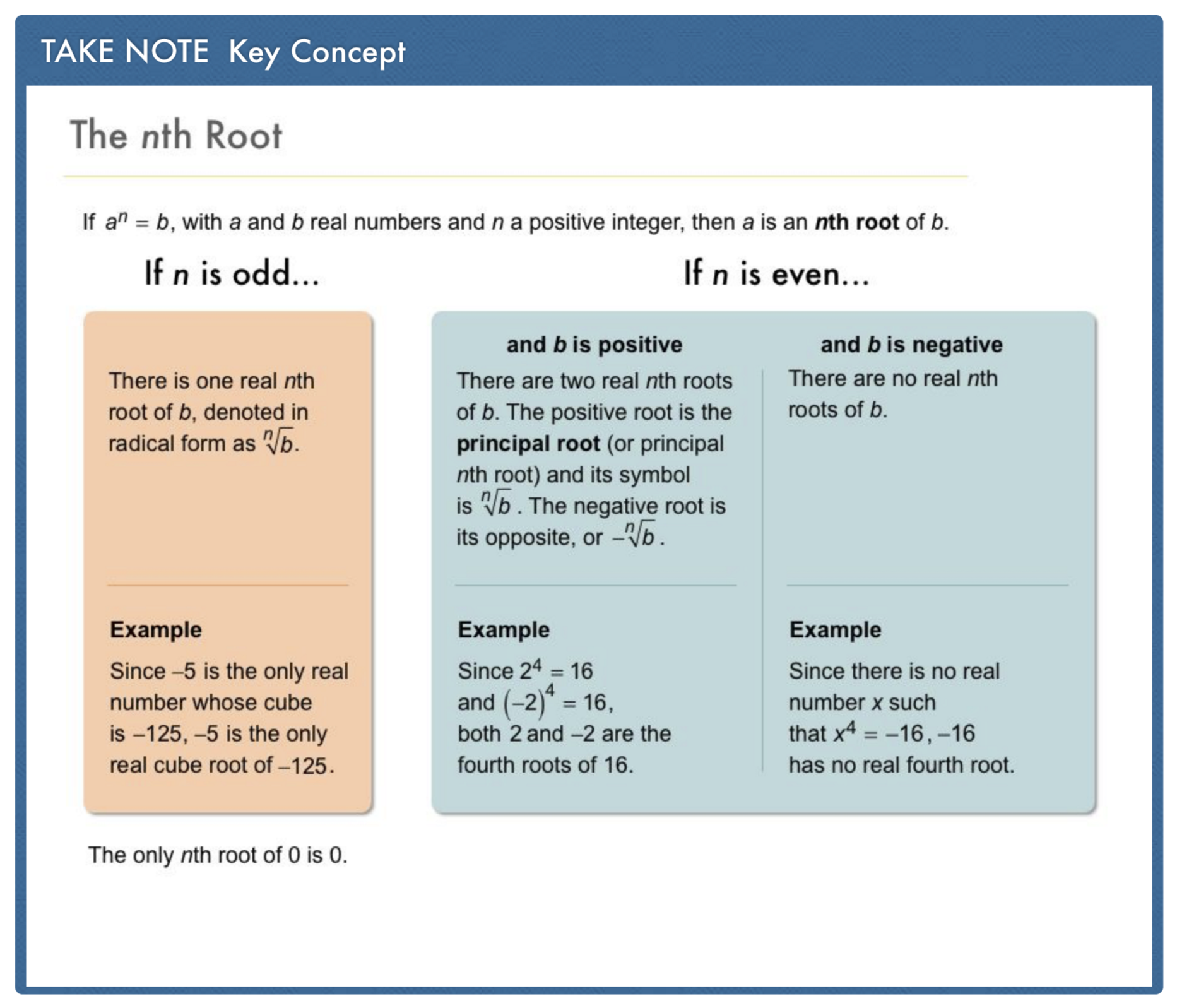2
10 pts
Problem 1 Got It? What are the fifth roots of 0, -1, and 32?
• -32
• -2
• -1
• no real root
• 0
• 1
• 2
• 32
• Fifth root of 0
• Fifth root of -1
• Fifth root of 32
3
10 pts
Problem 1 Got It? What are the real square roots of the following?

• -0.1
• -36/121
• -6/11
• no real root
• 0
• 0.1
• 6/11
• 36/121
• Real square root(s) of

• Real square root(s) of

• Real square root(s) of

4
10 pts
Problem 1 Got It? Reasoning: Explain why a negative real number b has no real nth roots if n is even.
By the rational root theorem and since b can be written as the following polynomial:
it follows that the only rational roots of b will be the factors of b over the factors of 0, which equal 0.
By Descartes' Rule of Signs and since b can be written as the following polynomial with no sign changes:

it follows that there can be at most 0 real nth roots.
Any negative number squared is a positive number. Therefore, there can be no real nth roots (where n is even) for a negative number b.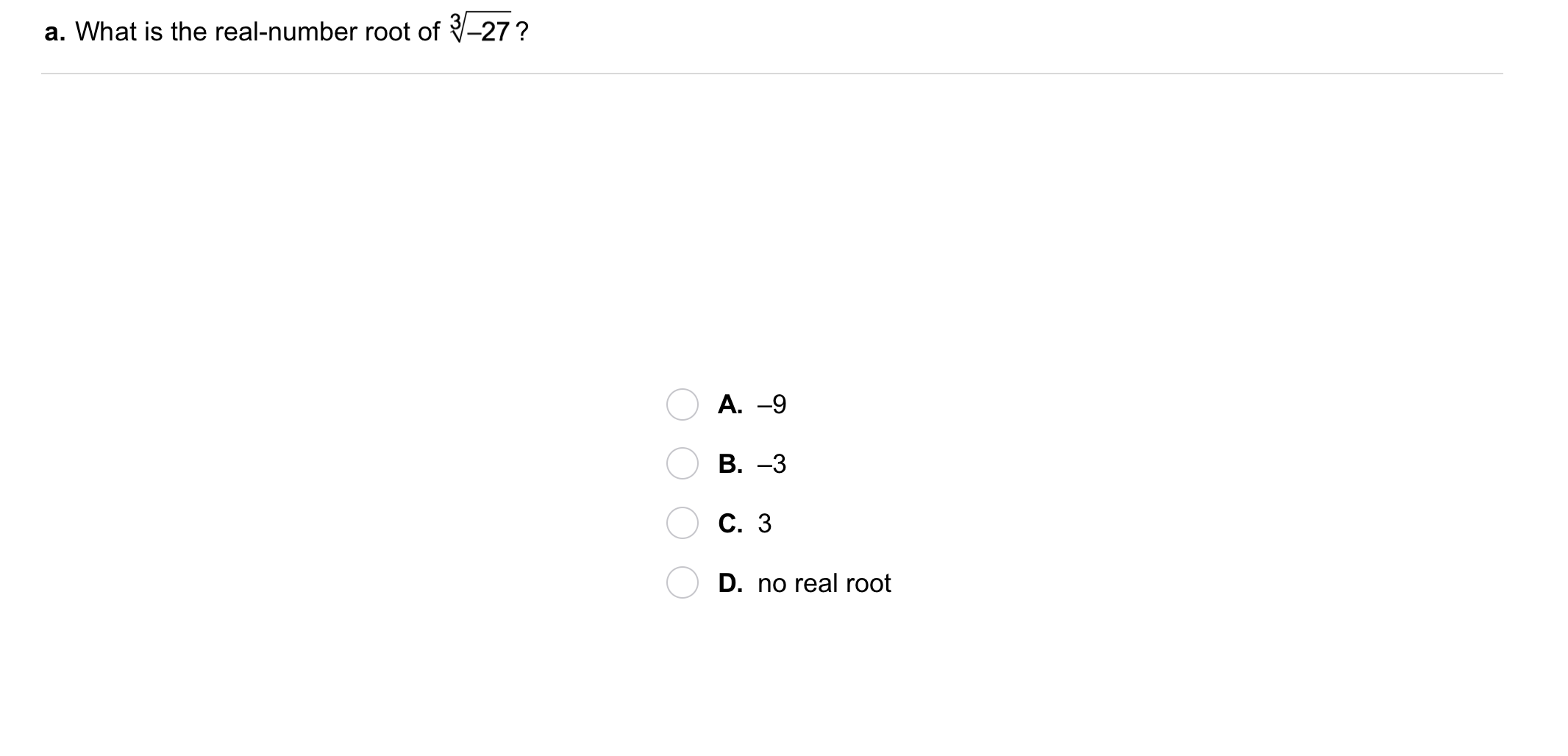5
5
10 pts
Problem 2 Got It?
A
B
C
D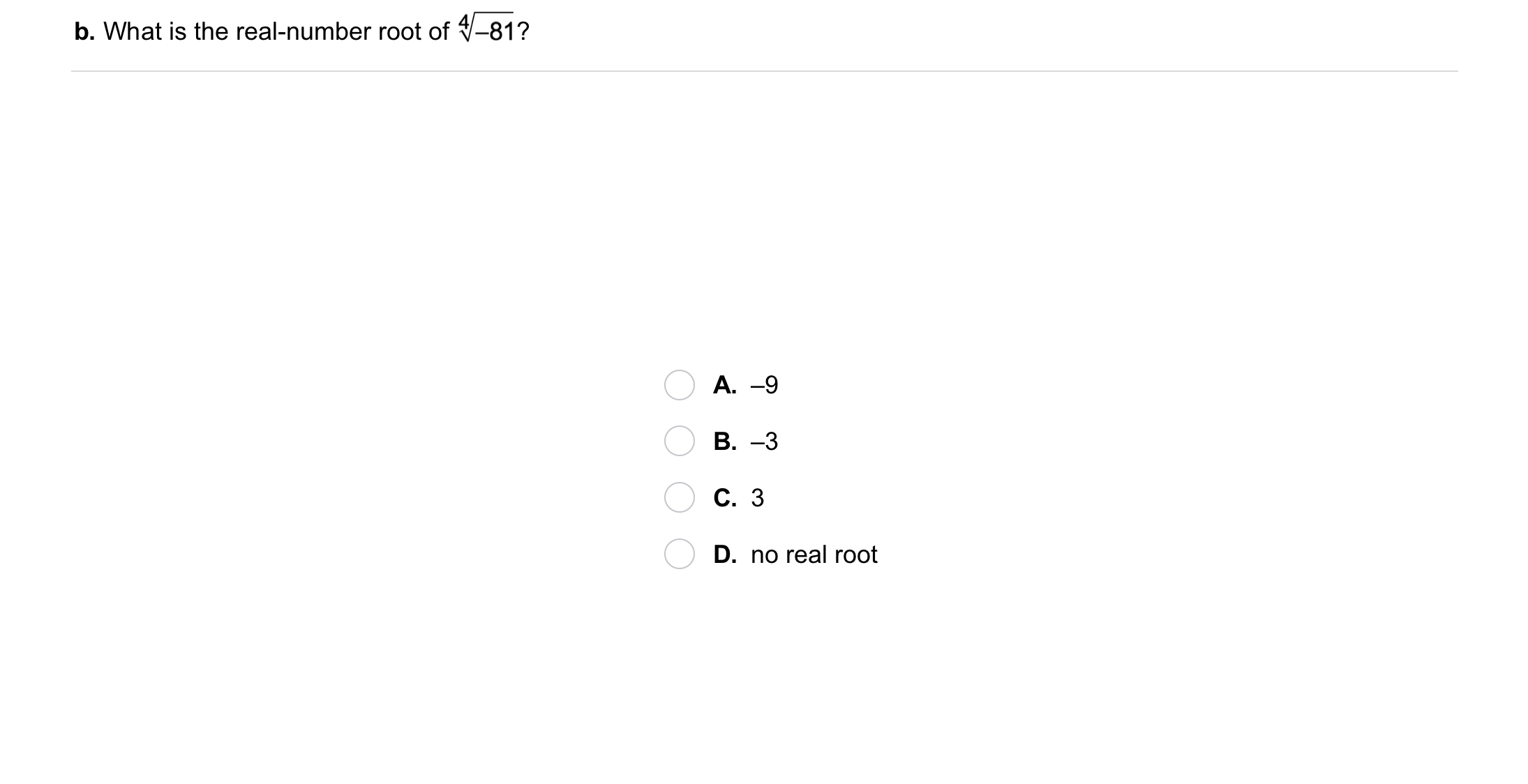6
6
10 pts
Problem 2 Got It?
A
B
C
D7
7
10 pts
Problem 2 Got It?
A
B
C
D8
8
10 pts
Problem 2 Got It?
A
B
C
D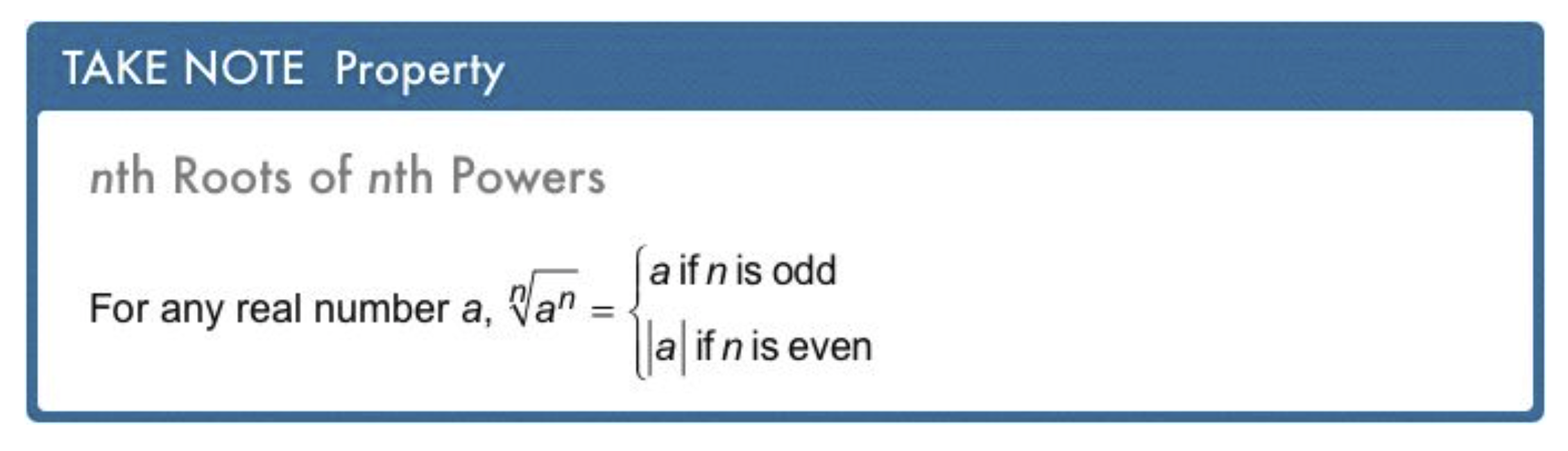9
9
10 pts
Problem 3 Got It?
A
B
C
D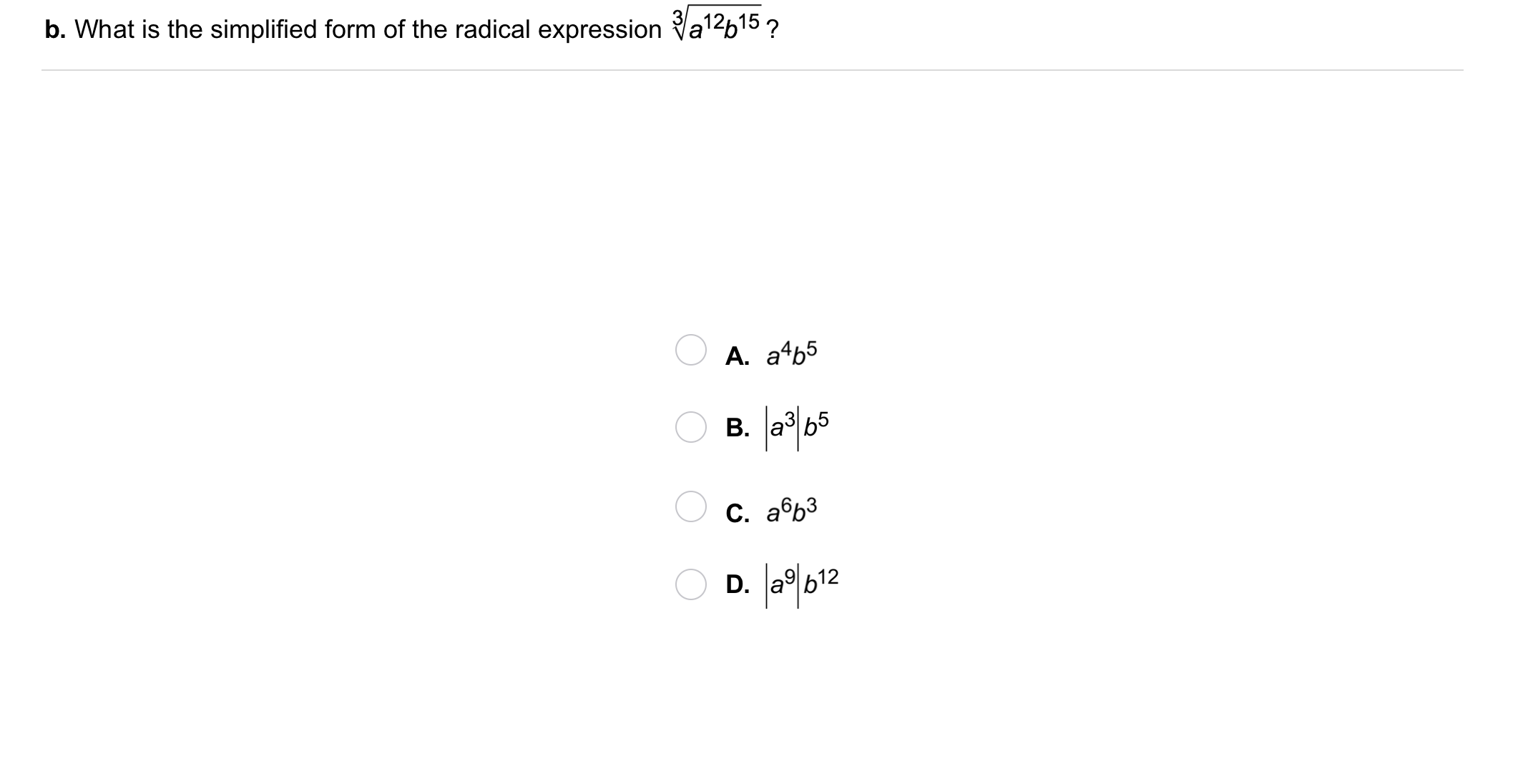10
10
10 pts
Problem 3 Got It?
A
B
C
D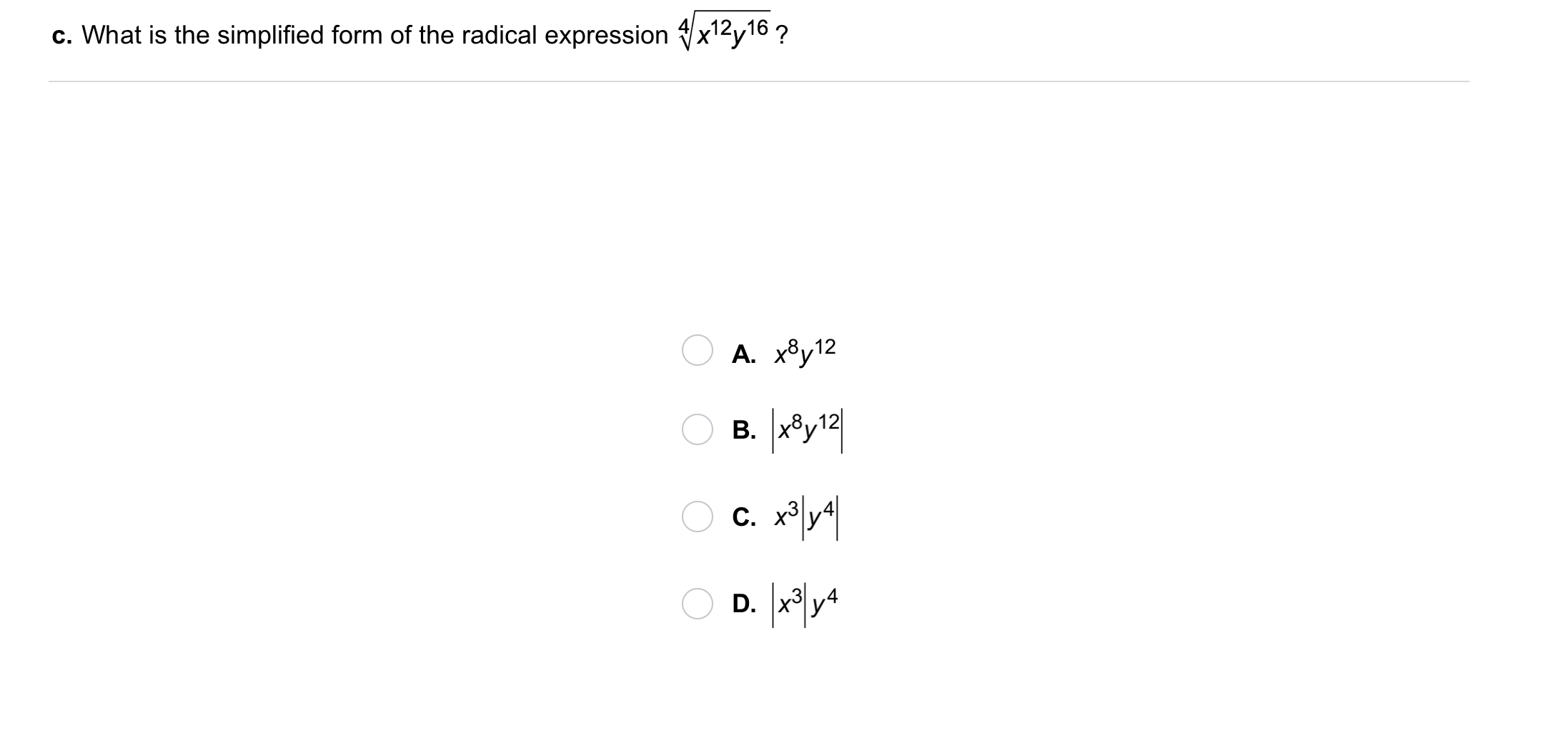11
11
10 pts
Problem 3 Got It?
A
B
C
D12
12
10 pts
Problem 4 Got It? Academics: Some teachers adjust test scores when a test is difficult. One teacher's formula for adjusting scores is show below, where A is the adjusted score and R is the raw score.
What are the adjusted scores for raw scores of 0 and 100?
A
B
C
D13
13
10 pts
What are all the real square roots of 25?
A
B
C
D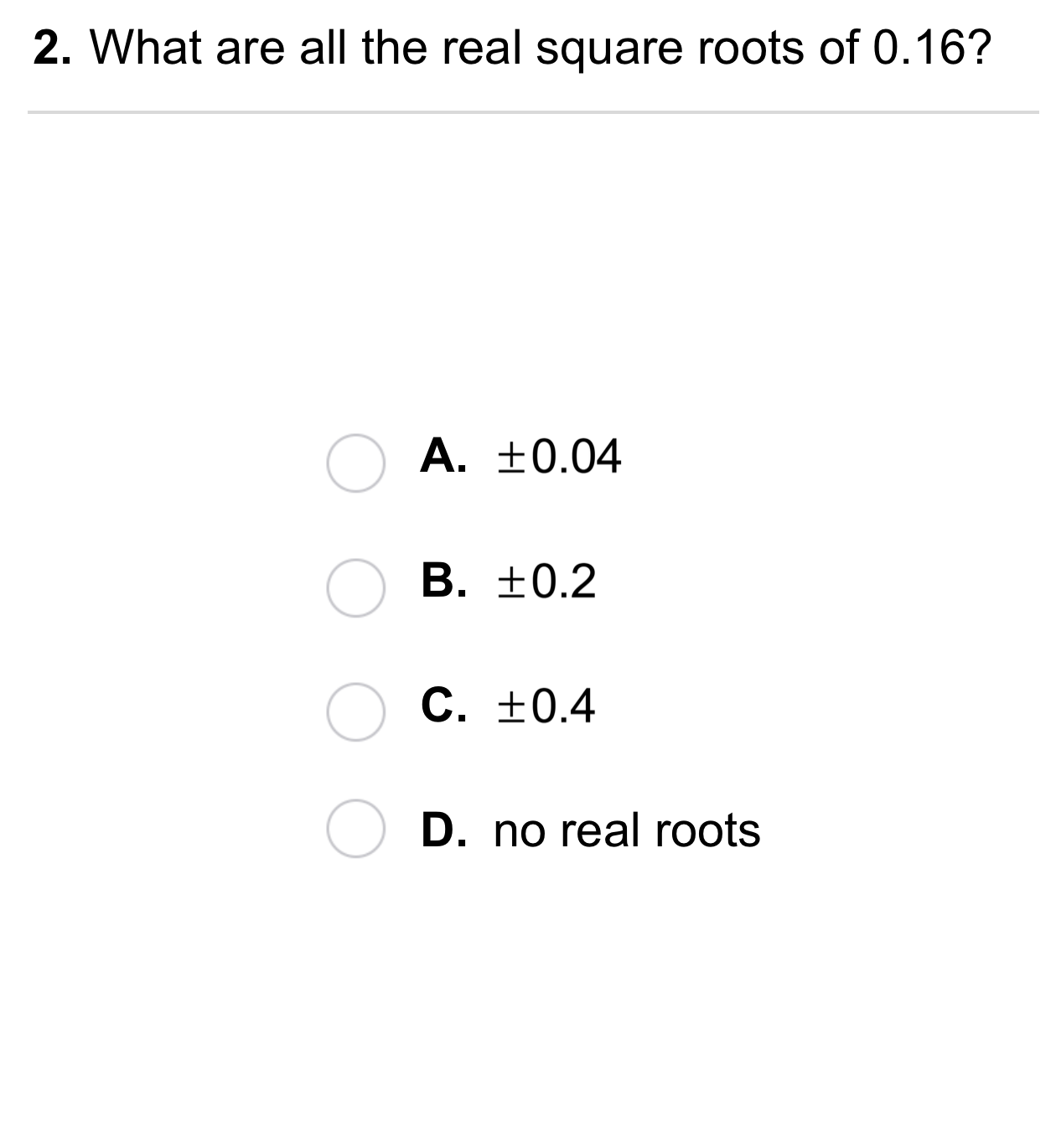14
14
10 pts
What are all the real square roots of 0.16?
A
B
C
D15
15
10 pts
What are all the real square roots of -64?
A
B
C
D16
16
10 pts
Simplify the radical expression.

A
B
C
D17
17
10 pts
Simplify the radical expression.

A
B
C
D18
18
10 pts
Simplify the radical expression.

A
B
C
D19
10 pts
Error Analysis: A student said the only fourth root of 16 is 2. Describe her error.
21
10 pts
Reasoning: A number has only one real nth root. What can you conclude about the index n?22
10 pts
Review Lesson 5-9: Determine the cubic function that is obtained from the parent function y = after the stated sequence of transformations.

• translation up 3 units and left 2 units
• vertical stretch by a factor of 3 and translation right 2 units
• translation right 2 units and down 3 units
23
10 pts
Review Lesson 4-7: Write the common form of the quadratic formula.
Hint: It shoud be in this format: x = [insert correct rational expression here].
24
10 pts
Review Lesson 4-7: Use the Quadratic Formula to identify the solution(s) of each quadratic equation.
Some solutions may not be used.

• Solution(s) of:

• Solution(s) of:

• Solution(s) of:

25
10 pts
Review Lesson 1-3: Simplify the algebraic expression.

26
10 pts
Review Lesson 1-3: Simplify the algebraic expression.

27
10 pts
Review Lesson 1-3: Simplify the algebraic expression.28
10 pts
Vocabulary Review: Identify the exponent(s) in each expression.
• -3
• 0
• 2
• 4
• 5
• 7
• 12
• 21

29
10 pts
Vocabulary Review: Which expression represents five to the second power ?30
10 pts
Use Your Vocabulary: Match each number in the left column to each term in the right column that best describes it as part of the equation.

• 4
• 81
• 3
• root
• index